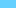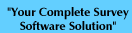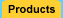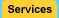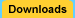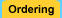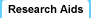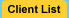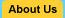Sample Size Calculator Significance Survey Design Correlation Interviewing Companies# Sample Size Formulas

Here are the formulas used in our Sample Size Calculator:

## Sample Size

 ss = Z2  * (p) * (1-p) c2

where:
 Z = Z value (e.g. 1.96 for 95% confidence level)  p = percentage picking a choice, expressed as decimal       (.5 used for sample size needed) c = confidence interval, expressed as decimal        (e.g., .04 = ±4)

## Correction for Finite Population

ss

new ss =

 1+ ss-1 pop

where:
 pop = population

 Survey Software Home | Products | Services | Downloads | Ordering Research Aids | Client List | About Us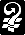Copyright © 2003  Creative Research Systems  (707) 765-1001  info@surveysystem.com